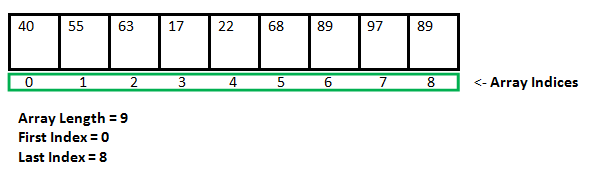Related Articles

# Arrays and Strings in C++

• Last Updated : 07 May, 2020

Arrays

An array in C or C++ is a collection of items stored at contiguous memory locations and elements can be accessed randomly using indices of an array. They are used to store similar types of elements as in the data type must be the same for all elements. They can be used to store the collection of primitive data types such as int, float, double, char, etc of any particular type. To add to it, an array in C or C++ can store derived data types such as the structures, pointers, etc.
There are two types of arrays:

• One Dimensional Array
• Multi Dimensional Array

One Dimensional Array: A one dimensional array is a collection of same data types. 1-D array is declared as:

```data_type variable_name[size]

data_type is the type of array, like int, float, char, etc.
variable_name is the name of the array.
size is the length of the array which is fixed.
```

Note: The location of the array elements depends upon the data type we use.

Below is the illustration of the array:Below is the program to illustrate the traversal of the array:

 `// C++ program to illustrate the traversal``// of the array``#include "iostream"``using` `namespace` `std;`` ` `// Function to illustrate traversal in arr[]``void` `traverseArray(``int` `arr[], ``int` `N)``{`` ` `    ``// Iterate from [1, N-1] and print``    ``// the element at that index``    ``for` `(``int` `i = 0; i < N; i++) {``        ``cout << arr[i] << ``' '``;``    ``}``}`` ` `// Driver Code``int` `main()``{`` ` `    ``// Given array``    ``int` `arr[] = { 1, 2, 3, 4 };`` ` `    ``// Size of the array``    ``int` `N = ``sizeof``(arr) / ``sizeof``(arr);`` ` `    ``// Function call``    ``traverseArray(arr, N);``}`
Output:
```1 2 3 4
```

MultiDimensional Array: A multidimensional array is also known as array of arrays. Generally, we use a two-dimensional array. It is also known as the matrix. We use two indices to traverse the rows and columns of the 2D array. It is declared as:

```data_type variable_name[N][M]

data_type is the type of array, like int, float, char, etc.
variable_name is the name of the array.
N is the number of rows.
M is the number of columns.
```

Below is the program to illustrate the traversal of the 2D array:

 `// C++ program to illustrate the traversal``// of the 2D array``#include "iostream"``using` `namespace` `std;`` ` `const` `int` `N = 2;``const` `int` `M = 2;`` ` `// Function to illustrate traversal in arr[][]``void` `traverse2DArray(``int` `arr[][M], ``int` `N)``{`` ` `    ``// Iterate from [1, N-1] and print``    ``// the element at that index``    ``for` `(``int` `i = 0; i < N; i++) {``        ``for` `(``int` `j = 0; j < M; j++) {``            ``cout << arr[i][j] << ``' '``;``        ``}``        ``cout << endl;``    ``}``}`` ` `// Driver Code``int` `main()``{`` ` `    ``// Given array``    ``int` `arr[][M] = { { 1, 2 }, { 3, 4 } };`` ` `    ``// Function call``    ``traverse2DArray(arr, N);`` ` `    ``return` `0;``}`
Output:
```1 2
3 4
```

Strings

C++ string class internally uses character array to store character but all memory management, allocation, and null termination are handled by string class itself that is why it is easy to use. For example it is declared as:

```char str[] = "GeeksforGeeks"
```

Below is the program to illustrate the traversal in the string:

 `// C++ program to illustrate the``// traversal of string``#include "iostream"``using` `namespace` `std;`` ` `// Function to illustrate traversal``// in string``void` `traverseString(``char` `str[])``{``    ``int` `i = 0;`` ` `    ``// Iterate till we found '\0'``    ``while` `(str[i] != ``'\0'``) {``        ``printf``(``"%c "``, str[i]);``        ``i++;``    ``}``}`` ` `// Driver Code``int` `main()``{`` ` `    ``// Given string``    ``char` `str[] = ``"GeekforGeeks"``;`` ` `    ``// Function call``    ``traverseString(str);`` ` `    ``return` `0;``}`
Output:
```G e e k f o r G e e k s
```

The string data_type in C++ provides various functionality of string manipulation. They are:

1. strcpy(): It is used to copy characters from one string to another string.
2. strcat(): It is used to add the two given strings.
3. strlen(): It is used to find the length of the given string.
4. strcmp(): It is used to compare the two given string.

Below is the program to illustrate the above functions:

 `// C++ program to illustrate functions``// of string manipulation``#include "iostream"``#include "string.h"``using` `namespace` `std;`` ` `// Driver Code``int` `main()``{`` ` `    ``// Given two string``    ``char` `str1 = ``"GeekforGeeks"``;``    ``char` `str2 = ``"HelloGeek"``;`` ` `    ``// To get the length of the string``    ``// use strlen() function``    ``int` `x = ``strlen``(str1);`` ` `    ``cout << ``"Length of string "` `<< str1``         ``<< ``" is "` `<< x << endl;`` ` `    ``cout << endl;`` ` `    ``// To compare the two string str1``    ``// and str2 use strcmp() function``    ``int` `result = ``strcmp``(str1, str2);`` ` `    ``// If result is 0 then str1 and str2``    ``// are equals``    ``if` `(result == 0) {``        ``cout << ``"String "` `<< str1``             ``<< ``" and String "` `<< str2``             ``<< ``" are equal."` `<< endl;``    ``}``    ``else` `{`` ` `        ``cout << ``"String "` `<< str1``             ``<< ``" and String "` `<< str2``             ``<< ``" are not equal."` `<< endl;``    ``}`` ` `    ``cout << endl;`` ` `    ``cout << ``"String str1 before: "``         ``<< str1 << endl;`` ` `    ``// Use strcpy() to copy character``    ``// from one string to another``    ``strcpy``(str1, str2);`` ` `    ``cout << ``"String str1 after: "``         ``<< str1 << endl;`` ` `    ``cout << endl;`` ` `    ``return` `0;``}`
Output:
```Length of string GeekforGeeks is 12

String GeekforGeeks and String HelloGeek are not equal.

String str1 before: GeekforGeeks
String str1 after: HelloGeek
```

Attention reader! Don’t stop learning now. Get hold of all the important DSA concepts with the DSA Self Paced Course at a student-friendly price and become industry ready.  To complete your preparation from learning a language to DS Algo and many more,  please refer Complete Interview Preparation Course.

In case you wish to attend live classes with experts, please refer DSA Live Classes for Working Professionals and Competitive Programming Live for Students.

My Personal Notes arrow_drop_up